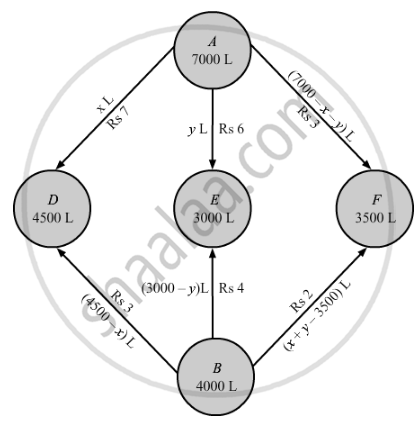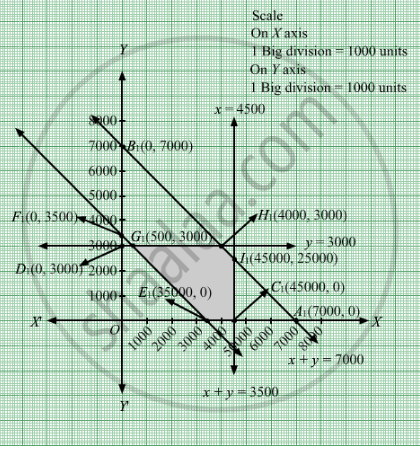# An Oil Company Has Two Depots, a and B, with Capacities of 7000 Litres and 4000 Litres Respectively. - Mathematics

Sum

An oil company has two depots, A and B, with capacities of 7000 litres and 4000 litres respectively. The company is to supply oil to three petrol pumps, DEF whose requirements are 4500, 3000 and 3500 litres respectively. The distance (in km) between the depots and petrol pumps is given in the following table:
Figure
Assuming that the transportation cost per km is Rs 1.00 per litre, how should the delivery be scheduled in order that the transportation cost is minimum?

#### Solution

Let x and y litres of oil be supplied from A to the petrol pumps D and E. Then, (7000 − − y) L will be supplied from A to petrol pump F.

The requirement at petrol pump D is 4500 L. Since, L are transported from depot A, the remaining (4500 −x) L will be transported from petrol pump B.

Similarly, (3000 − y) L and [3500 − (7000 − − y)] L i.e. (x + y − 3500) L will be transported from depot B to petrol pump E and F. respectively.

The given problem can be represented diagrammatically as follows.Since, quantity of oil are non-negative quantities.Therefore,

x ≥0 , y ≥ 0, and (7000 - x - y ) ≥ 0

⇒ x ≥ 0 , y ≥ 0, and x + y ≥ 7000

4500 - x ≥ 0 , 3000 - y ≥ 0 , and x + y -3500 ≥ 0

⇒ x ≤ 4500, y ≤ 3000, and x + y  ≥ 3500

Cost of transporting 10 L of petrol = Re 1

Cost of transporting 1 L of petrol =  $Rs \frac{1}{10}$

Therefore, total transportation cost is given by,

z = 7/10 xx x+6/10 y + 3/10 (7000 - x -y ) + 3/10 (4500 - x) + 4/10 ( 3000 - y ) + 2/10( x + y - 3500)

= 0.3x + 0.1y + 3950

The problem can be formulated as follows.

Minimize Z = 0.3x + 0.1y + 3950

subject to the constraints,

$x + y \leq 7000$
$x \leq 4500$
$y \leq 3000$
$x + y \geq 3500$
$x, y \geq 0$

First we will convert inequations into equations as follows:
x + y = 7000, x = 4500, y = 3000, y = 3500, x = 0 and y = 0

Region represented by x + y ≤ 7000:
The line x + y = 7000 meets the coordinate axes at A1(7000, 0) and  $B_1 \left( 0, 7000 \right)$ respectively. By joining these points we obtain the line x + y = 7000 . Clearly (0,0) satisfies the x + y = 7000 . So, the region which contains the origin represents the solution set of the inequation x + y ≤ 7000.

Region represented by x ​ ≤ 4500:
The line ​x = 4500 is the line passes through C1(4500, 0) and is parallel to Y axis. The region to the left of the line x = 4500 will satisfy the inequation
x ​ ≤ 4500.

Region represented by y ​ ≤ 3000:
The line ​y = 3000 is the line passes through D1(0, 3000) and is parallel to X axis. The region below the  the line y = 3000 will satisfy the inequation
y ​ ≤ 3000.

Region represented by x + y ≥ 3500:
The line x + y = 7000 meets the coordinate axes at E1(3500, 0) and $F_1 \left( 0, 3500 \right)$ respectively. By joining these points we obtain the line
x + y = 3500 . Clearly (0,0) satisfies the x + y = 3500. So, the region which contains the origin represents the solution set of the inequation x + y ≥ 3500.

Region represented by ≥ 0 and y ≥ 0:
Since, every point in the first quadrant satisfies these inequations. So, the first quadrant is the region represented by the inequations x ≥ 0, and y ≥ 0.

The feasible region determined by the system of constraints x + y ≤ 7000, x ​ ≤ 4500, y​ ≤ 3000, x + y ≥ 3500, x ≥ 0 and y ≥ 0 are as follows.The corner points of the feasible region are E1(3500, 0), C1(4500, 0), I1(4500, 2500), H1(4000, 3000), and G1(500, 3000).

The values of Z at these corner points are as follows.

 Corner point Z = 0.3x + 0.1y + 3950 E1(3500, 0) 5000 C1(4500, 0) 5300 I1(4500, 2500) 5550 H1(4000, 3000) 5450 G1(500, 3000) 4400

The minimum value of Z is 4400 at G1(500, 3000).

Thus, the oil supplied from depot A is 500 L, 3000 L, and 3500 L and from depot B is 4000 L, 0 L, and 0 L to petrol pumps DE, and F respectively.

The minimum transportation cost is Rs 4400.

Concept: Graphical Method of Solving Linear Programming Problems
Is there an error in this question or solution?

#### APPEARS IN

RD Sharma Class 12 Maths
Chapter 30 Linear programming
Exercise 30.4 | Q 37 | Page 55